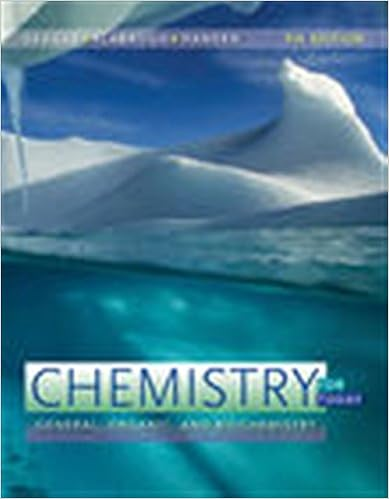# 2070-E2-ZincIodide-120904 - EXPERIMENT 2 Determination of...

• Lab Report
• stbp2
• 4

This preview shows page 1 - 2 out of 4 pages.

##### We have textbook solutions for you!
The document you are viewing contains questions related to this textbook.The document you are viewing contains questions related to this textbook.
Chapter 6 / Exercise 6.1
Chemistry for Today: General, Organic, and Biochemistry
Seager/SlabaughExpert Verified
2070 – Fall 2012 2-1 EXPERIMENT 2 Determination of Chemical Formulae: The Reaction of Zinc and Iodine Objective: In successfully completing this lab you will: synthesize and purify zinc iodide by reacting elemental zinc and iodine employ relative mass to determine the empirical formula for zinc iodide evaluate the validity of the Law of Mass Conservation investigate chemical reversibility by decomposing a compound (zinc iodide) back into its constituent elements (zinc and iodine, respectively) Introduction: When two elements react, they combine to form compounds with integer atom ratios. Although the individual atoms are too small to see and count, the simplest chemical formula (i.e. empirical formula) can be determined by keeping track of the mass of materials that react to form the compound. From the mass ratio of reactants, mole ratios between elements in a compound can be determined using the relative molar masses found on the periodic table. This atom bookkeeping is called stoichiometry, from the Greek stoikheion (element) and metron (to measure). In this experiment, zinc iodide will be synthesized by combining granular zinc metal with elemental iodine dissolved in a slightly acidic aqueous solution. The masses of iodine and zinc that react will be measured, from which the mole ratio, mol I/mol Zn , can be calculated and the empirical formula, Zn x I y determined. If accurate data are obtained, the empirical results for x & y will scale to those for simple integers, from which we can find the accepted values for x & y . There are at least two approaches to determine the stoichiometry of this reaction: (1) analyze a sample of pure zinc iodide to determine the masses of each element present in the compound; (2) synthesize zinc iodide by reacting a known mass of iodine with an excess of zinc and determine the masses of the product obtained as well as the leftover zinc. In this experiment, you will use the second approach. Because zinc is an insoluble solid and the reaction product is soluble in water, the product can be easily separated from unreacted zinc by decantation and washing. Moreover, if the masses of all materials are measured before and after the reaction, the amount of each present in the product can be determined. By
##### We have textbook solutions for you!
The document you are viewing contains questions related to this textbook.The document you are viewing contains questions related to this textbook.
Chapter 6 / Exercise 6.1
Chemistry for Today: General, Organic, and Biochemistry
Seager/SlabaughExpert Verified
•••